﻿ 基于核相关滤波器的目标跟踪算法
«上一篇文章快速检索 高级检索

 应用科技2017, Vol. 44Issue (3): 48-53  DOI: 10.11991/yykj.2016050130

### 引用本文ZHU Xiaoxiong, JIANG Jiahe. Visual tracking algorithm based on kernelized correlation filters[J]. Applied Science and Technology, 2017, 44(3), 48-53. DOI: 10.11991/yykj.201605013.### 文章历史

Visual tracking algorithm based on kernelized correlation filters
ZHU Xiaoxiong, JIANG Jiahe
National Defense Key Laboratory, Beihang University, Beijing 100191, China
Abstract: To address the disturbance caused by object deformation, occlusion and out-of-plane rotation in visual tracking, this paper proposed a new kernelized correlation filtering algorithm on the basis of color name and space features by improving the traditional kernelized correlation filters (KCF) tracking algorithm on the aspects of feature extraction mode and model updating scheme.In order to verify the effectiveness of the algorithm, 38 color video sequences were selected in visual benchmark datasets for verifying the tracking algorithm.In addition, the paper compared the performance of the algorithm with other competitive visual tracking algorithms such as KCF, structured output tracking with kernel (Struck), tracking-learning-detection (TLD) and sparsity-based collaborative model (SCM).Results show the proposed algorithm not only has the best performance, but also is more adaptive to tracking challenges such as deformation, occlusion and out-of-plane rotation.
Key words: visual tracking    kernelized correlation filters    color name space features    feature extraction    model updating scheme    deformation    occlusion    out-of-plane rotation

1 基于检测的目标跟踪系统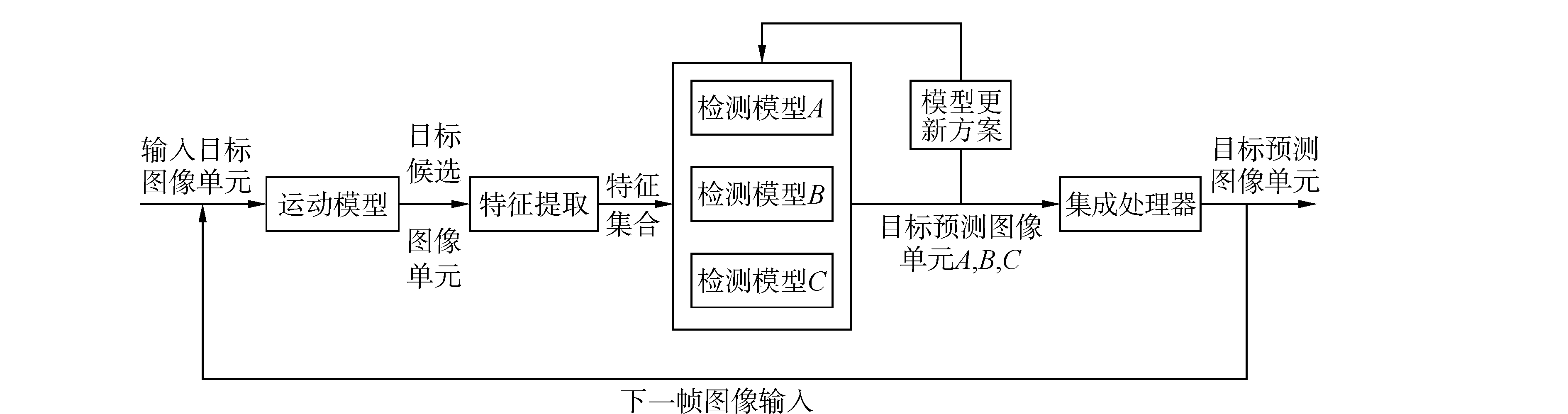图 1 基于检测的目标跟踪系统框架

2 核相关滤波器算法分析

2.1 运动模型

 $\mathit{\boldsymbol{P}}{\rm{ = }}\left[\begin{array}{l} 0\;\;\;0\;\;\;0\;\; \cdots \;\;\;1\\ 1\;\;\;0\;\;\;0\;\;\; \cdots \;\;\;0\\ 0\;\;\;1\;\;\;0\;\;\; \cdots \;\;\;0\\ \vdots \;\;\;\; \vdots \;\;\;\; \vdots \;\;\;\;\;\;\;\;\;\; \vdots \\ 0\;\;\;0\;\;\; \cdots \;\;\;1\;\;\;0 \end{array} \right]$ (1)

 $\mathit{\boldsymbol{X}} = C\left( x \right) = \left[\begin{array}{l} {x_1}\;\;\;{x_2}\;\;\;{x_3}\;\;\; \cdots \;\;\;{x_n}\\ {x_n}\;\;\;{x_1}\;\;\;{x_2}\;\;\; \cdots \;\;\;{x_{n-1}}\\ {x_{n-1}}\;\;{x_n}\;\;\;{x_1}\;\; \cdots \;\;\;{x_{n-2}}\\ \vdots \;\;\;\;\;\;\; \vdots \;\;\;\;\; \vdots \;\;\;\;\;\;\;\;\;\;\;\;\; \vdots \\ {x_2}\;\;\;\;{x_3}\;\;\;\;{x_4}\;\; \cdots \;\;\;{x_1} \end{array} \right]$ (2)

2.2 特征提取

1) 原始像素特征：通过将图像转换到一定的尺寸，然后将其转换成灰度图像，以每个像素的灰度值作为图像的特征。

2) HOG特征：HOG特征首次由Dalal等提出，用于进行行人检测。HOG特征通过计算和统计图像局部区域的梯度方向直方图来提取图像特征。

3) CN特征：CN特征首先由Danelljan等引入到目标跟踪算法当中，这种特征将RGB空间的图像信息转换到11维空间的颜色描述特征中。同时，将颜色名称空间映射到10维的标准正交子空间。这种映射在减少CN特征维度的同时，也将CN特征进行了聚集。

2.3 检测模型

 $\mathop {\min }\limits_w {\sum\limits_i {{{\left( {f\left( {{x_i}} \right)-{y_i}} \right)}^2} + \lambda \left\| \mathit{\boldsymbol{w}} \right\|} ^2}$ (3)

 $\mathit{\boldsymbol{w}} = \sum\limits_i {{\alpha _i}\varphi \left( {{x_i}} \right)}$ (4)

 $\mathit{\boldsymbol{\alpha = }}{\left( {\mathit{\boldsymbol{K}} + \lambda \mathit{\boldsymbol{I}}} \right)^{-1}}\mathit{\boldsymbol{y}}$ (5)

 $\mathit{\boldsymbol{\hat \alpha = }}\frac{{\mathit{\boldsymbol{\hat y}}}}{{\mathit{\boldsymbol{\hat k + \lambda }}}}$ (6)

 $f\left( z \right) = \left\langle {\mathit{\boldsymbol{w}}, \varphi \left( z \right)} \right\rangle = \sum\limits_i {{\alpha _i}k\left( {z, {x_i}} \right)}$ (7)

2.4 模型更新方案

1) 传统KCF算法中给出以下模型更新方案。

 ${\mathit{\boldsymbol{x}}_t} = \left( {1-\eta } \right){\mathit{\boldsymbol{x}}_{t-1}} + \eta {\mathit{\boldsymbol{x}}_t}$ (8)
 ${\mathit{\boldsymbol{\alpha }}_t} = \left( {1-\eta } \right){\mathit{\boldsymbol{\alpha }}_{t-1}} + \eta {\mathit{\boldsymbol{\alpha }}_t}$ (9)

 $\varepsilon = \sum\limits_{j = 1}^{\rm{t}} {\left( {\sum\limits_i {{{\left| {\left\langle {{\mathit{\boldsymbol{w}}^j}, \varphi \left( {x_i^j} \right)} \right\rangle-y_i^j} \right|}^2} + \lambda {{\left\| {{\mathit{\boldsymbol{w}}^j}} \right\|}^2}} } \right)}$ (10)

 ${\mathit{\boldsymbol{\hat \alpha }}^t} = \frac{{\sum\limits_{j = 1}^t {{{\hat y}^j}{{\mathit{\boldsymbol{\hat K}}}^j}} }}{{\sum\limits_{j = 1}^t {{{\mathit{\boldsymbol{\hat K}}}^j}\left( {{{\mathit{\boldsymbol{\hat K}}}^j} + \lambda } \right)} }}$ (11)

2) 改进后的模型更新方法

 $\mathit{\boldsymbol{\hat \alpha }}_{{\rm{num}}}^t = \left( {1-\eta } \right)\mathit{\boldsymbol{\hat \alpha }}_{{\rm{num}}}^{t-1} + \eta {\hat y^t}{\mathit{\boldsymbol{\hat K}}^t}$ (12)
 $\mathit{\boldsymbol{\hat \alpha }}_{{\rm{den}}}^t = \left( {1-\eta } \right)\mathit{\boldsymbol{\hat \alpha }}_{{\rm{den}}}^{t-1} + \eta {\mathit{\boldsymbol{\hat K}}^t}\left( {{{\mathit{\boldsymbol{\hat K}}}^t} + \lambda } \right)$ (13)
 ${\mathit{\boldsymbol{x}}^t} = \left( {1-\eta } \right){\mathit{\boldsymbol{x}}^{t-1}} + \eta {\mathit{\boldsymbol{x}}^t}$ (14)

3 实验分析

3.1 模型更新方案比较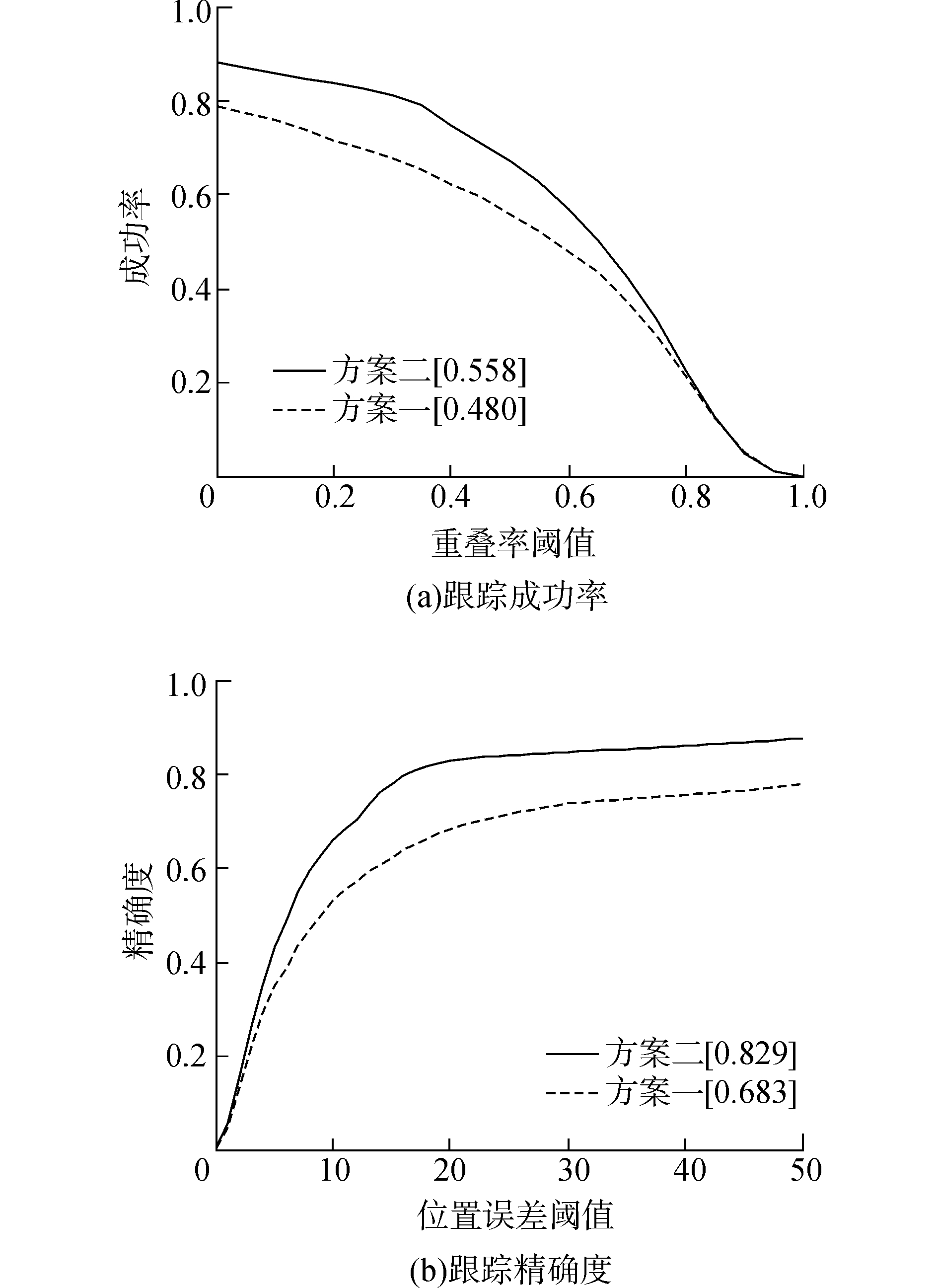图 2 不同模型更新方案下跟踪算法的结果

3.2 特征提取比较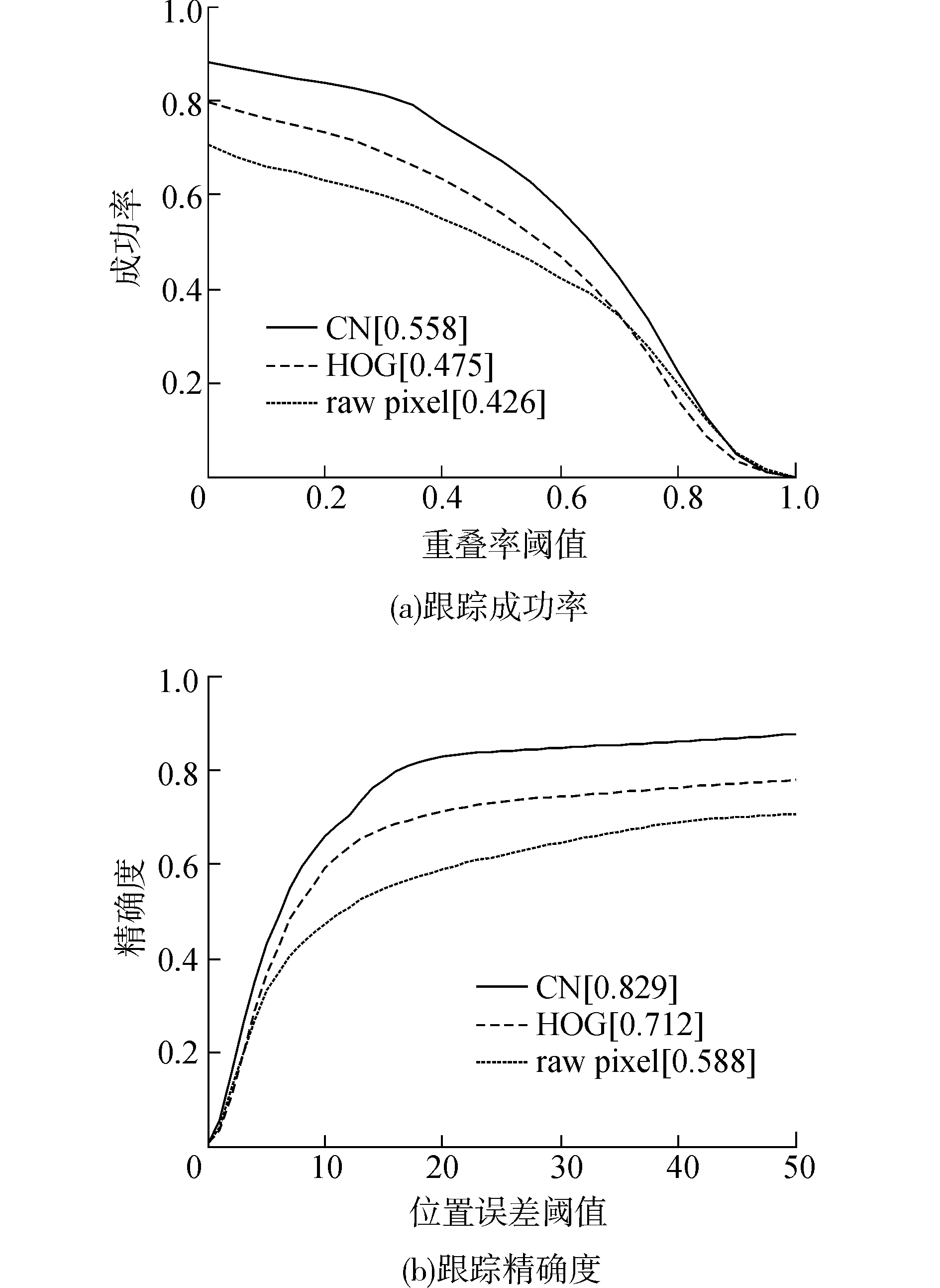图 3 不同特征提取下的跟踪算法的结果

3.3 改进的核相关滤波跟踪算法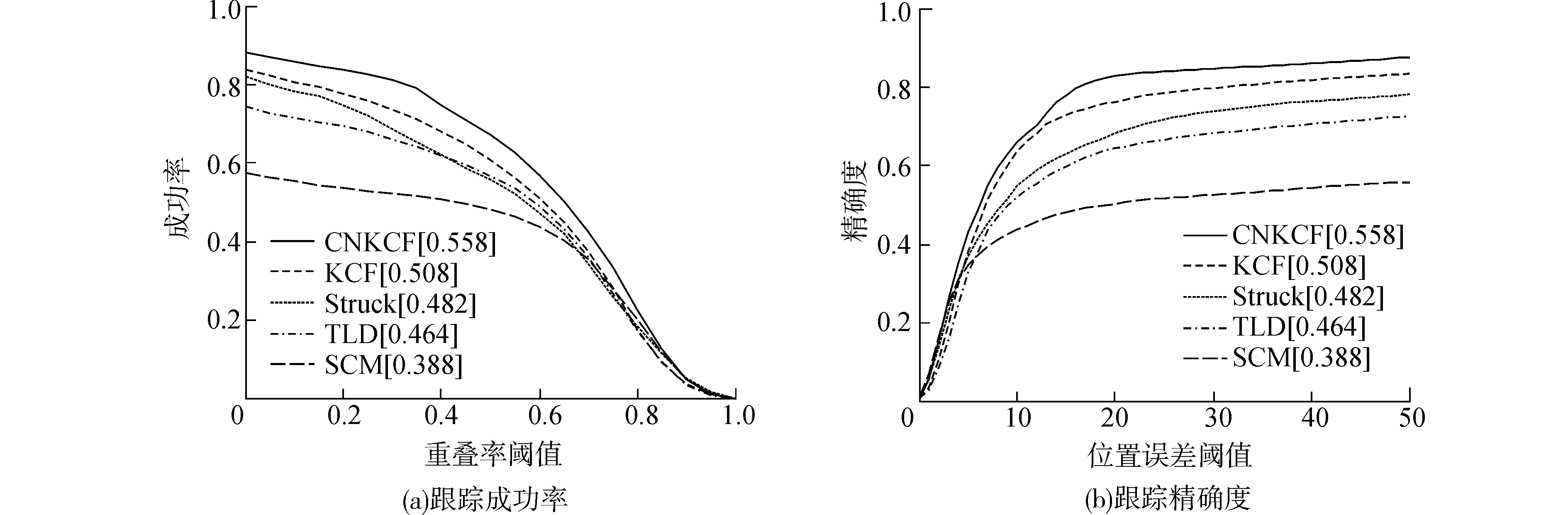图 4 不同跟踪算法的跟踪结果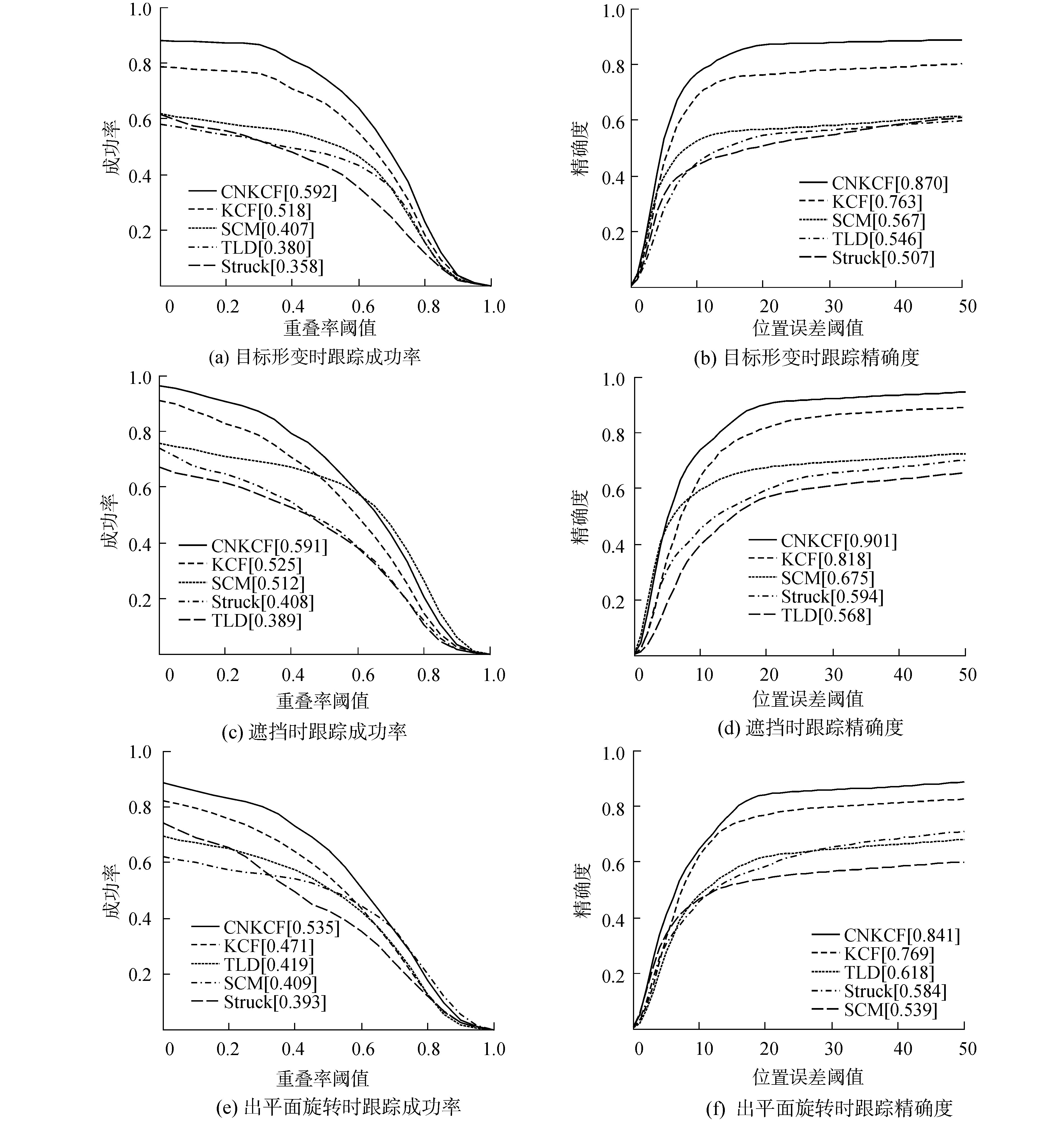图 5 不同跟踪算法在干扰条件下的跟踪结果
4 结论

1) 选取合适的特征描述子和模型更新方案能够极大地提高跟踪算法的性能；

2) 不同特征对各种干扰条件的抗干扰能力不同。

  钟必能, 陈雁, 沈映菊, 等. 在线机器学习跟踪算法的研究进展[J]. 华侨大学学报:自然科学版, 2014, 35(1): 41-46. (0)  LIU Qi, ZHAO Xiaoguang, HOU Zengguang. Survey of single-target visual tracking methods based on online learning[J]. IET computer vision, 2014, 8(5): 419-428. DOI:10.1049/iet-cvi.2013.0134 (0)  HARE S, SAFFARI A, TORR P H S. Structured output tracking with kernels[C]//Proceedings of the 2011 IEEE International Conference on Computer Vision. Barcelona:IEEE, 2011:263-270. (0)  KALAL Z, MIKOLAJCZYK K, MATAS J. Tracking-learning-detection[J]. IEEE transactions on pattern analysis and machine intelligence, 2012, 34(7): 1409-1422. DOI:10.1109/TPAMI.2011.239 (0)  ZHANG Kaihua, ZHANG Lei, YANG M H. Real-time compressive tracking[C]//ECCV 2012:Proceedings of the 12th European Conference on Computer Vision. Berlin:Springer, 2012:864-877. (0)  HENRIQUES J F, CASEIRO R, MARTINS P, el al. Exploiting the circulant structure of tracking-by-detection with kernels[C]//ECCV 2012:Proceedings of the 12th European Conference on Computer Vision. Berlin:Springer, 2012:702-715. (0)  HENRIQUES J F, CASEIRO R, MARTINS P, et al. High-speed tracking with kernelized correlation filters[J]. IEEE transactions on pattern analysis and machine intelligence, 2015, 37(3): 583-596. DOI:10.1109/TPAMI.2014.2345390 (0)  WANG Naiyan, SHI Jianping, YEUNG D Y, et al. Understanding and diagnosing visual tracking systems[C]//2015 IEEE International Conference on Computer Vision. Santiago:IEEE, 2015:3101-3109. (0)  VAN DE WEIJER J, SCHMID C, VERBEEK J, et al. Learning color names for real-world applications[J]. IEEE transactions on image processing, 2009, 18(7): 1512-1523. DOI:10.1109/TIP.2009.2019809 (0)  DALAL N, TRIGGS B. Histograms of oriented gradients for human detection[C]//IEEE Computer Society Conference on Computer Vision and Pattern Recognition. Santiago:IEEE, 2005:886-893. (0)  DANELLJAN M, KHAN F S, FELSBERG M, et al. Adaptive color attributes for real-time visual tracking[C]//Proceedings of the 2014 IEEE Conference on Computer Vision and Pattern recognition. Columbus, OH:IEEE, 2014:1090-1097. (0)  RIFKIN R, YEO G, POGGIO T. Regularized least-squares classification[J]. Nato Science Series Sub Series Ⅲ Computer and Systems Science, 2003: 131-154. (0)  YANG M H, LIM J, WU Yi. Online object tracking:a benchmark[C]//Proceedings of the 2013 IEEE Conference on Computer Vision and Pattern Recognition. Portland, OR:IEEE, 2013:2411-2418. (0)  ZHONG Wei, LU Huchuan, YANG M H. Robust object tracking via sparsity-based collaborative model[C]//Proceedings of 2012 IEEE Conference on Computer Vision and Pattern Recognition. Providence, RI:IEEE, 2012:1838-1845. (0)